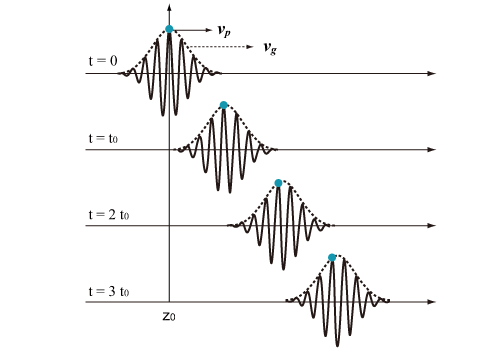Light is electromagnetic wave. A propagation velocity of equivalent phase plane of electromagnetic wave is called as the phase velocity, vp. In a pulse propagation, an energy transfer velocity of electromagnetic wave is called as group velocity, vg. For the electromagnetic (optical) pulse, the group velocity is a transfer velocity of envelope curve of a pulse

Fig.2.13 explains the phase velocity, vp, and the group velocity, vg, for vp<vg in the pulse propagation. the • mark in Fig.2.13 is added for descriptive purpose to understand the phase velocity without effort. The velocity of • mark corresponds to the phase velocity. It can be seen that the equivalent phase point travels with a relative delay to the envelope curve.Figure 2.13: Phase velocity and group velocity (vp < vg) during pulse propagation

The group velocity, vg, depends on the frequency, and dvg/dω does not equal to 0. This frequency dependency is called as group velocity dispersion (GVD). An ultrashort pulse propagation is largely affected by GVD.